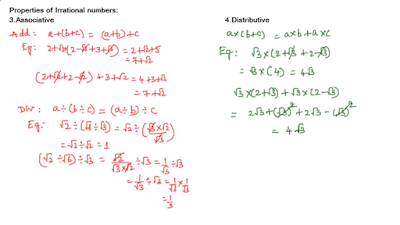Support us .Net Basics C# SQL ASP.NET Aarvi MVC Slides C# Programs Subscribe Download

### Number Systems - Properties of Irrational Numbers

Suggested Videos
Part 7 - Operations on real numbers - Text - Slides

Properties:
• Closure
• Commutative
• Associative
• Distributive
Let us prove the above properties over basic arithmetic operations (Addition, Subtraction, Multiplication and Division) by considering some examplesFig (1) Closure and Commutative property

From Figure 1, we can conclude that Irrational numbers are not closed under arithmetic operations. Addition and Multiplication satisfies associative property.Fig (2) Associative and Distributive properties

From figure 2, we can conclude that addition satisfies associative property, division is not satisfying this property. Similarly we can prove multiplication and subtraction associative property. Multiplication over addition satisfies distributive property.

Conclusion:
• Irrational numbers are not closed under basic arithmetic operations.
• Irrational numbers satisfy the commutative, associative and distributive laws for addition and multiplication.